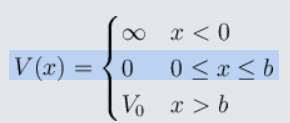# Quantum mechanics - several constant potentials

Eitan Levy
Homework Statement:
A particle with mass m is in a one dimensional potential, as seen below.

The wave function in $x<0$ is 0.

The wave function in $0<x<b$ is: $Asin(kx)$

The wave function in $x>b$ is: $Be^{-\alpha x}$

It is known that $k=3*10^{10}$ and $b=0.5333333*10^{-10}$

Find $\alpha$
Relevant Equations:
Schrodinger stationary equationWhat I tried to do was using the fact that the wave function should be continuous.

$Asin(kb)=Be^{-\alpha b}$

The derivative also should be continuous:

$kAcos(kb)=-\alpha Be^{-\alpha b}$

And the probability to find the particle in total should be 1:

$\int_0^b A^2sin^2(kx) dx + \int_b^{\infty} B^2e^{-2\alpha x} dx =1$

This set of equations is to hard to deal with, the equations should be solved with calculator only so I think I did something wrong.

Also, there may be a better way to approach this problem, but I'm not seeing it.

•Delta2

Homework Helper
Gold Member
2022 Award
$Asin(kb)=Be^{-\alpha b}$

$kAcos(kb)=-\alpha Be^{-\alpha b}$

$\int_0^b A^2sin^2(kx) dx + \int_b^{\infty} B^2e^{-2\alpha x} dx =1$
Hints: It's much easier than you think. You only need the first 2 equations.

•Delta2
Eitan Levy
Hints: It's much easier than you think. You only need the first 2 equations.

Homework Helper
Gold Member
2022 Award
I haven't done the calculation. How did you get that value?
Also, have you forgotten units or were there really none supplied in the original question?

Eitan Levy
I haven't done the calculation. How did you get that value?
Also, have you forgotten units or were there really none supplied in the original question?
I divided the first equation by the second:

$\frac{1}{k}tan(kb)=-\frac{1}{\alpha}$

And I plugged in the numbers.

No units were provided.

•Delta2 and Steve4Physics
Homework Helper
Gold Member
2022 Award
I divided the first equation by the second:

$\frac{1}{k}tan(kb)=-\frac{1}{\alpha}$

And I plugged in the numbers.

No units were provided.
Agreed. Well done! A couple of points:
1) You haven't correctly used standard form (mantissa in wrong range).
2) You have rounded the last digit incorrectly when giving the answer to 4 significant figures.

If working in SI units, what do you think the unit would be? (If I were handing this in, I would make a comment about the appropriate SI units below my answer.)

•Eitan Levy
Eitan Levy
Agreed. Well done! A couple of points:
1) You haven't correctly used standard form (mantissa in wrong range).
2) You have rounded the last digit incorrectly when giving the answer to 4 significant figures.

If working in SI units, what do you think the unit would be? (If I were handing this in, I would make a comment about the appropriate SI units below my answer.)
$(Angstram)^{-1}$

Homework Helper
Gold Member
2022 Award
$(Angstram)^{-1}$
The ångström (Å) (note spelling and capitalisation) is a bit old-fashioned, but is still used in some areas. It is not an SI unit.

1 Å = 10⁻¹⁰ m
That means
1 Å⁻¹ =10¹⁰ m⁻¹

I would have given the answer as:
α = 8.76x10⁸ (m⁻¹ assuming we are working in SI units)

EDIT: It's very poor practice to give values without units. The question should have given the units for k and b.